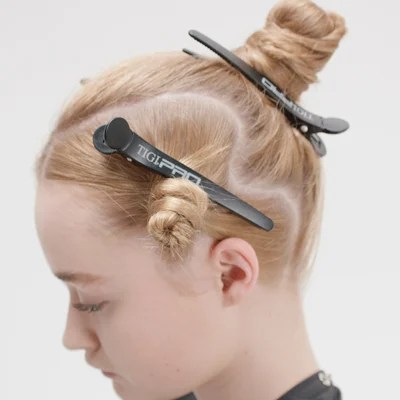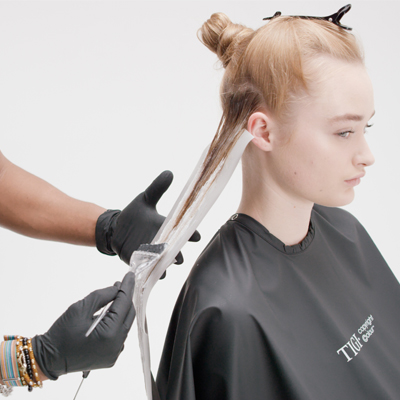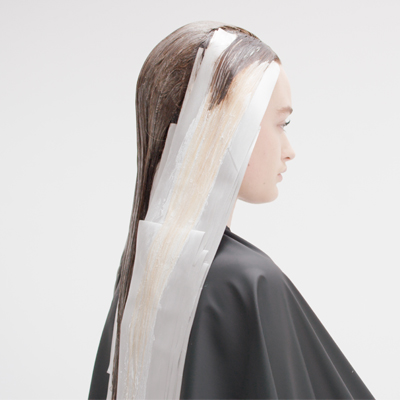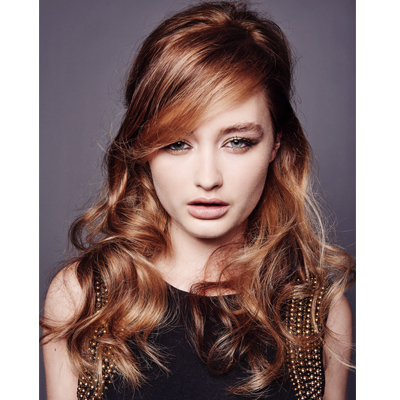Contour Lightening Technique from TIGI
November 19, 2015

# Contour Lightening Technique from TIGI

VIEW ALL PHOTOS VIEW SLIDESHOW
GET THE HOW-TO• Formula A

• Formula B

30g true light white + 45g activator 5vol/1.55%

• Formula C

60g gloss 9/32 + 90g activator 8.5vol/2.55%

• Formula D

40g gloss 9/32 + 60g activator 5vol/1.5%# Contour Lightening Technique from TIGI

Contouring with makeup has been around for a while now, but did you know that you can enhance facial structure with makeup, too? TIGI’s new Contour Lightening Technique uses three different lighter color variants to create a contouring effect, defining the shape of the hair and promoting shine. It’s how TIGI American Technical Director Richy Kandasamy created this stunning, honey-toned blend. Read on for the exclusive formula and application steps!

Manufacturer: TIGI, @tigiprofessionals

## COLOR FORMULAS• Formula A

• Formula B

30g true light white + 45g activator 5vol/1.55%

• Formula C

60g gloss 9/32 + 90g activator 8.5vol/2.55%

• Formula D

40g gloss 9/32 + 60g activator 5vol/1.5%

## HOW-TO STEPS•• Formula A

• Formula B

30g true light white + 45g activator 5vol/1.55%

• Formula C

60g gloss 9/32 + 90g activator 8.5vol/2.55%

• Formula D

40g gloss 9/32 + 60g activator 5vol/1.5%

1

Create a profile parting from the forehead, finishing at the top crown.

•• Formula A

• Formula B

30g true light white + 45g activator 5vol/1.55%

• Formula C

60g gloss 9/32 + 90g activator 8.5vol/2.55%

• Formula D

40g gloss 9/32 + 60g activator 5vol/1.5%

2

Start by taking an irregular C section, starting 2 inches back from the hairline, finishing at the nape area. Secure with a TIGI PRO Sectioning Clip.

•• Formula A

• Formula B

30g true light white + 45g activator 5vol/1.55%

• Formula C

60g gloss 9/32 + 90g activator 8.5vol/2.55%

• Formula D

40g gloss 9/32 + 60g activator 5vol/1.5%

3

Repeat the same process on the opposite side and secure with a TIGI PRO Sectioning Clip.

•• Formula A

• Formula B

30g true light white + 45g activator 5vol/1.55%

• Formula C

60g gloss 9/32 + 90g activator 8.5vol/2.55%

• Formula D

40g gloss 9/32 + 60g activator 5vol/1.5%

4

Through the back area, secure the remaining hair using a TIGI PRO Sectioning Clip. In total, this creates three sections. Put on protective gloves.

•• Formula A

• Formula B

30g true light white + 45g activator 5vol/1.55%

• Formula C

60g gloss 9/32 + 90g activator 8.5vol/2.55%

• Formula D

40g gloss 9/32 + 60g activator 5vol/1.5%

5

Start the application through the C section. Take a 1-inch deep irregular parting. Apply Formula 1 from the new growth area through to the midlengths. With a free-hand blending technique, apply Formula 2 to the lengths and ends. Isolate using a TIGI copyright©olour meche strip.

•• Formula A

• Formula B

30g true light white + 45g activator 5vol/1.55%

• Formula C

60g gloss 9/32 + 90g activator 8.5vol/2.55%

• Formula D

40g gloss 9/32 + 60g activator 5vol/1.5%

6

From the third slice, apply Formula 1 from the new growth area through to the mid-lengths. With a free-hand blending technique, apply Formula 3 to the lengths and ends. Isolate using a TIGI copyright©olour meche strip, repeat the same on the opposite side.

•• Formula A

• Formula B

30g true light white + 45g activator 5vol/1.55%

• Formula C

60g gloss 9/32 + 90g activator 8.5vol/2.55%

• Formula D

40g gloss 9/32 + 60g activator 5vol/1.5%

7

Progress into the last remaining section, take horizontal partings and apply Formula 1 from the new growth area through to the lengths and ends.

•• Formula A

• Formula B

30g true light white + 45g activator 5vol/1.55%

• Formula C

60g gloss 9/32 + 90g activator 8.5vol/2.55%

• Formula D

40g gloss 9/32 + 60g activator 5vol/1.5%

8

Continue in the same way until the section is completed.

•• Formula A

• Formula B

30g true light white + 45g activator 5vol/1.55%

• Formula C

60g gloss 9/32 + 90g activator 8.5vol/2.55%

• Formula D

40g gloss 9/32 + 60g activator 5vol/1.5%

9

Process  for 20 minutes, then emulsify, rinse and remove using Catwalk Headshot Shampoo and Conditioner. Then tone the prelightened sections through the sides using Formula 4. Process visually for up to 20 minutes, then emulsify, rinse and remove, using Catwalk Headshot Shampoo and Conditioner.

•• Formula A

• Formula B

30g true light white + 45g activator 5vol/1.55%

• Formula C

60g gloss 9/32 + 90g activator 8.5vol/2.55%

• Formula D

40g gloss 9/32 + 60g activator 5vol/1.5%

10
•• Formula A

• Formula B

30g true light white + 45g activator 5vol/1.55%

• Formula C

60g gloss 9/32 + 90g activator 8.5vol/2.55%

• Formula D

40g gloss 9/32 + 60g activator 5vol/1.5%

11